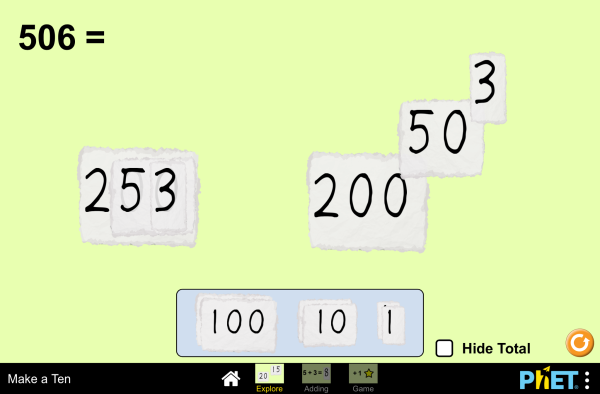Make a Ten_湊到 10下載嵌入 關閉 嵌入模擬教學執行複本 利用 HTML 來嵌入模擬教學執行複本。您可以在 HTML 中改變已嵌入模擬教學的寬度及高度。 嵌入啓動模擬教學之圖樣

• Place Value
• Subtraction
• 算術

描述

Add numbers by making tens. Break apart and combine numbers while focusing on place value. Use the adding screen to add any two numbers. Use the game screen to apply your make-a-ten strategies!

HTML5 版本之翻譯：

學習目標

• Improve understanding of place value by using different sizes of papers for ones, tens, and hundreds.
• Develop mental math strategies when taking apart and putting together numbers.
• Use the “make a ten” strategy when counting and doing addition.
• Develop a mental model of the basic properties of numbers including commutativity, associativity, and closure.

標準對齊

共用核心 - 數學

1.NBT.B.2
Understand that the two digits of a two-digit number represent amounts of tens and ones. Understand the following as special cases:
1.NBT.B.2a
10 can be thought of as a bundle of ten ones — called a "ten."
1.NBT.B.2b
The numbers from 11 to 19 are composed of a ten and one, two, three, four, five, six, seven, eight, or nine ones.
1.NBT.B.2c
The numbers 10, 20, 30, 40, 50, 60, 70, 80, 90 refer to one, two, three, four, five, six, seven, eight, or nine tens (and 0 ones).
1.NBT.C.4
Add within 100, including adding a two-digit number and a one-digit number, and adding a two-digit number and a multiple of 10, using concrete models or drawings and strategies based on place value, properties of operations, and/or the relationship between addition and subtraction; relate the strategy to a written method and explain the reasoning used. Understand that in adding two-digit numbers, one adds tens and tens, ones and ones; and sometimes it is necessary to compose a ten.
1.NBT.C.5
Given a two-digit number, mentally find 10 more or 10 less than the number, without having to count; explain the reasoning used.
1.NBT.C.6
Subtract multiples of 10 in the range 10-90 from multiples of 10 in the range 10-90 (positive or zero differences), using concrete models or drawings and strategies based on place value, properties of operations, and/or the relationship between addition and subtraction; relate the strategy to a written method and explain the reasoning used.
1.OA.B.3
Apply properties of operations as strategies to add and subtract.2 Examples: If 8 + 3 = 11 is known, then 3 + 8 = 11 is also known. (Commutative property of addition.) To add 2 + 6 + 4, the second two numbers can be added to make a ten, so 2 + 6 + 4 = 2 + 10 = 12. (Associative property of addition.)
1.OA.C.6
Add and subtract within 20, demonstrating fluency for addition and subtraction within 10. Use strategies such as counting on; making ten (e.g., 8 + 6 = 8 + 2 + 4 = 10 + 4 = 14); decomposing a number leading to a ten (e.g., 13 - 4 = 13 - 3 - 1 = 10 - 1 = 9); using the relationship between addition and subtraction (e.g., knowing that 8 + 4 = 12, one knows 12 - 8 = 4); and creating equivalent but easier or known sums (e.g., adding 6 + 7 by creating the known equivalent 6 + 6 + 1 = 12 + 1 = 13).
2.NBT.B.5
Fluently add and subtract within 100 using strategies based on place value, properties of operations, and/or the relationship between addition and subtraction.
2.NBT.B.6
Add up to four two-digit numbers using strategies based on place value and properties of operations.
2.NBT.B.7
Add and subtract within 1000, using concrete models or drawings and strategies based on place value, properties of operations, and/or the relationship between addition and subtraction; relate the strategy to a written method. Understand that in adding or subtracting three-digit numbers, one adds or subtracts hundreds and hundreds, tens and tens, ones and ones; and sometimes it is necessary to compose or decompose tens or hundreds.
2.NBT.B.8
Mentally add 10 or 100 to a given number 100-900, and mentally subtract 10 or 100 from a given number 100-900.
2.OA.C.3
Determine whether a group of objects (up to 20) has an odd or even number of members, e.g., by pairing objects or counting them by 2s; write an equation to express an even number as a sum of two equal addends.
2.OA.C.4
Use addition to find the total number of objects arranged in rectangular arrays with up to 5 rows and up to 5 columns; write an equation to express the total as a sum of equal addends.

教學提示模擬教學的控制概觀、模型簡化與引導學生思考 ( PDF ).

老師提供的活動

Preguntas de razonamiento para todas las simulaciones HTML5Diana López 大學-進階

K-5

Double-Digit Addition Susan O'Reilly K-5 指引 數學
Standard Adding Algorithm Demonstration Beth Stade K-5 展示 數學
Intervention and Targeted Practice Tips Beth Stade K-5 其它

Number Talks Beth Stade K-5 其它

Jogo da Adição com Números Naturais no OA "Tire um 10" Renata Passos Machado Vieira e Francisco Regis Vieira Alves K-5 其它

Operações Aritméticas Fundamentais Georgyana Cidrão; Francisco Régis Vieira Alves K-5 實驗室

Quadro Valor e Lugar - QVL Carolina Silveira 國中 實驗室 數學

Serbian 全部 СрпскиДопуни до 10

The new HTML5 sims can run on iPads and Chromebooks, as well as PC, Mac, and Linux systems.

iOS 11+ Safari

Android:
Not officially supported. If you are using the HTML5 sims on Android, we recommend using the latest version of Google Chrome.

Chromebook:
The HTML5 and Flash PhET sims are supported on all Chromebooks.
Chromebook compatible sims

Windows Systems:

Macintosh Systems:
macOS 10.9.5+, Safari 9+, latest version of Chrome.

Linux Systems:

• Amanda McGarry (co-lead designer)
• Jonathan Olson (developer)
• Sharfudeen Ashraf (developer)
• Ariel Paul
• Kathy Perkins
• Mariah Hermsmeyer (graphic arts)
• almond-0.2.9.js
• base64-js-1.2.0.js
• easing-equations-r12
• FileSaver-b8054a2.js
• font-awesome-4.5.0
• game-up-camera-1.0.0.js
• jama-1.0.2
• jquery-2.1.0.js
• lodash-2.4.1.js
• pegjs-0.7.0.js
• seedrandom-2.4.2.js
• text-2.0.12.js
• TextEncoderLite-3c9f6f0.js
• Tween-r12.js